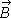# Magnetic Field Induced Current

## Homework Statement

A uniform magnetic fieldis perpendicular to the plane of a circular loop of diameter 18 cm formed from wire of diameter5.0 mm and resistivity 1.69 ✕ 10−8 Ω · m. At what rate must the magnitude ofchange to induce a 10 A current in the loop

## Homework Equations

Φ = ∫ B ⋅ dA (in vector form)
φ= BA
ε= -dΦ/dt
ε=IR
R= ρL/A

## The Attempt at a Solution

Current is given, so I fould the Emf using ε=IR
ε= (10) x ((1.69E-8)(2π⋅0.09))/(π⋅0.0052)) = 0.001217

Then using the value obtained for ε,
0.001217 = dΦ/dt
Φ= BA

0.001217⋅A = dB/dt

wouldn't dB/dT be the rate that B is changing?

mfb
Mentor
wouldn't dB/dT be the rate that B is changing?
It is, but the units are not Ampere. In general, working with units helps to find most errors.
Be careful with diameter and radius of the wire.

•PhysicsInNJ
Oh I see, my mistake was actually I used diameter instead of radius. Thank you!